11.3 Graphing with intercepts  (Page 3/4)

 Page 3 / 4

Graph using the intercepts: $y=3x.$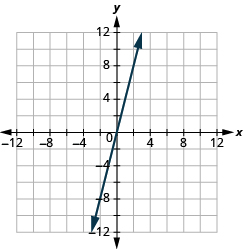Graph using the intercepts: $y=-x.$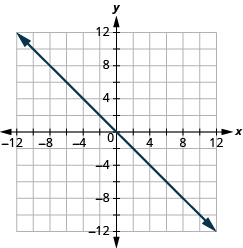Choose the most convenient method to graph a line

While we could graph any linear equation    by plotting points, it may not always be the most convenient method. This table shows six of equations we’ve graphed in this chapter, and the methods we used to graph them.

Equation Method
#1 $y=2x+1$ Plotting points
#2 $y=\frac{1}{2}x+3$ Plotting points
#3 $x=-7$ Vertical line
#4 $y=4$ Horizontal line
#5 $2x+y=6$ Intercepts
#6 $4x-3y=12$ Intercepts

What is it about the form of equation that can help us choose the most convenient method to graph its line?

Notice that in equations #1 and #2, y is isolated on one side of the equation, and its coefficient is 1. We found points by substituting values for x on the right side of the equation and then simplifying to get the corresponding y- values.

Equations #3 and #4 each have just one variable. Remember, in this kind of equation the value of that one variable is constant; it does not depend on the value of the other variable. Equations of this form have graphs that are vertical or horizontal lines.

In equations #5 and #6, both x and y are on the same side of the equation. These two equations are of the form $Ax+By=C$ . We substituted $y=0$ and $x=0$ to find the x- and y- intercepts, and then found a third point by choosing a value for x or y .

This leads to the following strategy for choosing the most convenient method to graph a line.

Choose the most convenient method to graph a line.

1. If the equation has only one variable. It is a vertical or horizontal line.
• $x=a$ is a vertical line passing through the $x\text{-axis}$ at $a$
• $y=b$ is a horizontal line passing through the $y\text{-axis}$ at $b.$
2. If $y$ is isolated on one side of the equation. Graph by plotting points.
• Choose any three values for $x$ and then solve for the corresponding $y\text{-}$ values.
3. If the equation is of the form $Ax+By=C,$ find the intercepts.
• Find the $x\text{-}$ and $y\text{-}$ intercepts and then a third point.

Identify the most convenient method to graph each line:

1. $\phantom{\rule{0.2em}{0ex}}y=-3\phantom{\rule{0.2em}{0ex}}$
2. $\phantom{\rule{0.2em}{0ex}}4x-6y=12\phantom{\rule{0.2em}{0ex}}$
3. $\phantom{\rule{0.2em}{0ex}}x=2\phantom{\rule{0.2em}{0ex}}$
4. $\phantom{\rule{0.2em}{0ex}}y=\frac{2}{5}x-1$

Solution

$\phantom{\rule{0.2em}{0ex}}y=-3$

This equation has only one variable, $y.$ Its graph is a horizontal line crossing the $y\text{-axis}$ at $-3.$

$\phantom{\rule{0.2em}{0ex}}4x-6y=12$

This equation is of the form $Ax+By=C.$ Find the intercepts and one more point.

$\phantom{\rule{0.2em}{0ex}}x=2$

There is only one variable, $x.$ The graph is a vertical line crossing the $x\text{-axis}$ at $2.$

$\phantom{\rule{0.2em}{0ex}}y=\frac{2}{5}x-1$

Since $y$ is isolated on the left side of the equation, it will be easiest to graph this line by plotting three points.

Identify the most convenient method to graph each line:

1. $\phantom{\rule{0.2em}{0ex}}3x+2y=12$
2. $\phantom{\rule{0.2em}{0ex}}y=4$
3. $\phantom{\rule{0.2em}{0ex}}y=\frac{1}{5}x-4$
4. $\phantom{\rule{0.2em}{0ex}}x=-7$
1. intercepts
2. horizontal line
3. plotting points
4. vertical line

Identify the most convenient method to graph each line:

1. $\phantom{\rule{0.2em}{0ex}}x=6$
2. $\phantom{\rule{0.2em}{0ex}}y=-\frac{3}{4}x+1$
3. $\phantom{\rule{0.2em}{0ex}}y=-8$
4. $\phantom{\rule{0.2em}{0ex}}4x-3y=-1$

1. vertical line
2. plotting points
3. horizontal line
4. intercepts

Key concepts

• Intercepts
• The x- intercept is the point, $\left(a,0\right)$ , where the graph crosses the x- axis. The x- intercept occurs when y is zero.
• The y- intercept is the point, $\left(0,b\right)$ , where the graph crosses the y- axis. The y- intercept occurs when y is zero.
• The x- intercept occurs when y is zero.
• The y- intercept occurs when x is zero.
• Find the x and y intercepts from the equation of a line
• To find the x- intercept of the line, let $y=0$ and solve for x .
• To find the y- intercept of the line, let $x=0$ and solve for y .
x y
0
0
• Graph a line using the intercepts
1. Find the x- and y- intercepts of the line.
• Let $y=0$ and solve for x.
• Let $x=0$ and solve for y.
2. Find a third solution to the equation.
3. Plot the three points and then check that they line up.
4. Draw the line.
• Choose the most convenient method to graph a line
1. Determine if the equation has only one variable. Then it is a vertical or horizontal line.
$x=a$ is a vertical line passing through the x- axis at a .
$y=b$ is a vertical line passing through the y- axis at b .
2. Determine if y is isolated on one side of the equation. The graph by plotting points.
Choose any three values for x and then solve for the corresponding y- values.
3. Determine if the equation is of the form $Ax+By=C$ , find the intercepts.
Find the x- and y- intercepts and then a third point.

Practice makes perfect

Identify the Intercepts on a Graph

In the following exercises, find the $x\text{-}$ and $y\text{-}$ intercepts.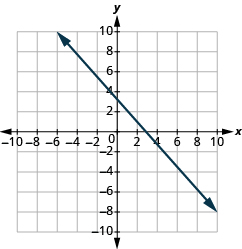(3,0),(0,3)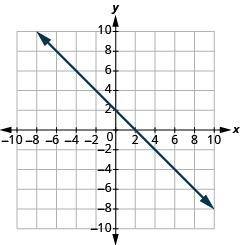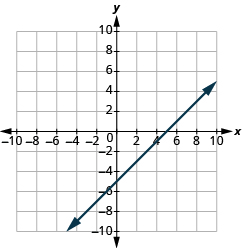(5,0),(0,−5)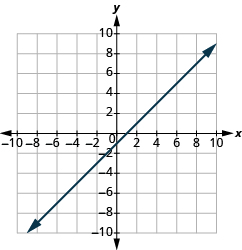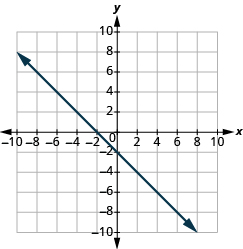(−2,0),(0,−2)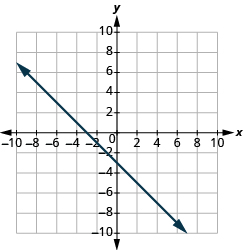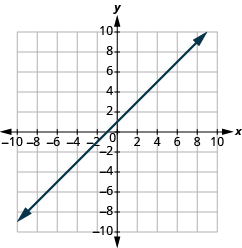(−1,0),(0,1)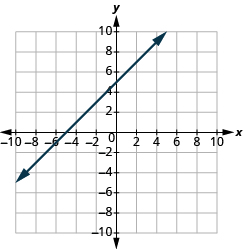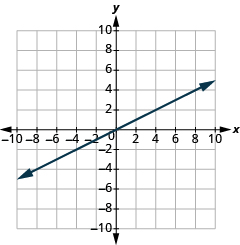(0,0)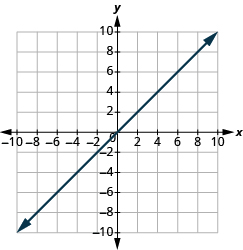Find the $x$ and $y$ Intercepts from an Equation of a Line

In the following exercises, find the intercepts.

$x+y=4$

(4,0),(0,4)

$x+y=3$

$x+y=-2$

(−2,0),(0,−2)

$x+y=-5$

$x-y=5$

(5,0),(0,−5)

$x-y=1$

$x-y=-3$

(−3,0),(0,3)

$x-y=-4$

$x+2y=8$

(8,0),(0,4)

$x+2y=10$

$3x+y=6$

(2,0),(0,6)

$3x+y=9$

$x-3y=12$

(12,0),(0,−4)

$x-2y=8$

$4x-y=8$

(2,0),(0,−8)

$5x-y=5$

$2x+5y=10$

(5,0),(0,2)

$2x+3y=6$

$3x-2y=12$

(4,0),(0,−6)

$3x-5y=30$

$y=\frac{1}{3}x-1$

(3,0),(0,−1)

$y=\frac{1}{4}x-1$

$y=\frac{1}{5}x+2$

(−10,0),(0,2)

$y=\frac{1}{3}x+4$

$y=3x$

(0,0)

$y=-2x$

$y=-4x$

(0,0)

$y=5x$

Graph a Line Using the Intercepts

In the following exercises, graph using the intercepts.

$-x+5y=10$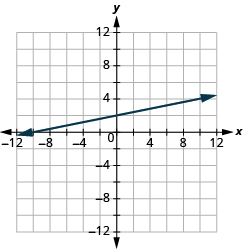$-x+4y=8$

$x+2y=4$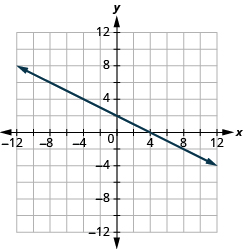$x+2y=6$

$x+y=2$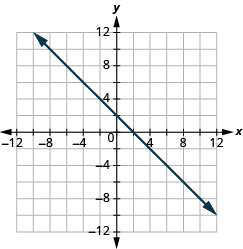$x+y=5$

$x+y=-3$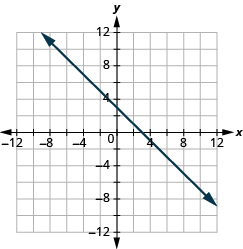$x+y=-1$

$x-y=1$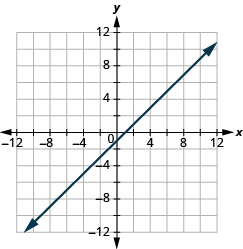$x-y=2$

$x-y=-4$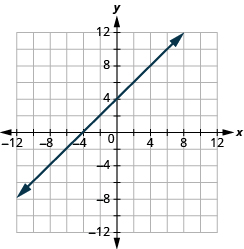$x-y=-3$

$4x+y=4$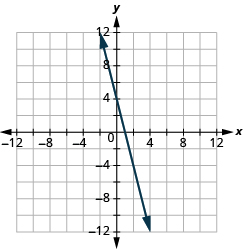$3x+y=3$

$3x-y=-6$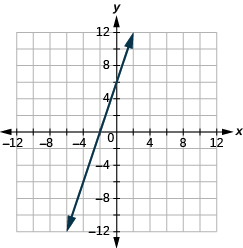$2x-y=-8$

$2x+4y=12$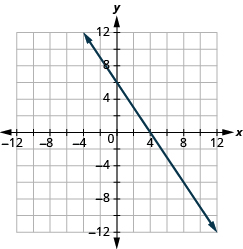$3x+2y=12$

$3x-2y=6$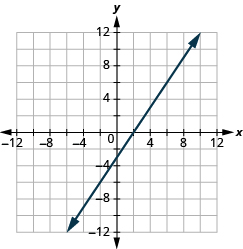$5x-2y=10$

$2x-5y=-20$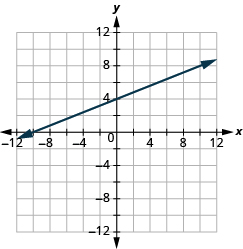$3x-4y=-12$

$y=-2x$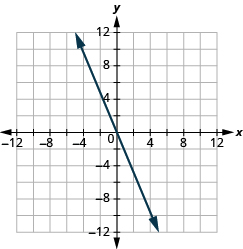$y=-4x$

$y=x$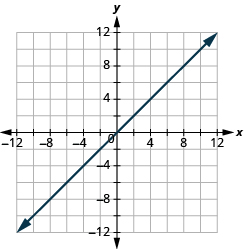$y=3x$

Choose the Most Convenient Method to Graph a Line

In the following exercises, identify the most convenient method to graph each line.

$x=2$

vertical line

$y=4$

$y=5$

horizontal line

$x=-3$

$y=-3x+4$

plotting points

$y=-5x+2$

$x-y=5$

intercepts

$x-y=1$

$y=\frac{2}{3}x-1$

plotting points

$y=\frac{4}{5}x-3$

$y=-3$

horizontal line

$y=-1$

$3x-2y=-12$

intercepts

$2x-5y=-10$

$y=-\frac{1}{4}x+3$

plotting points

$y=-\frac{1}{3}x+5$

Everyday math

Road trip Damien is driving from Chicago to Denver, a distance of $1,000$ miles. The $x\text{-axis}$ on the graph below shows the time in hours since Damien left Chicago. The $y\text{-axis}$ represents the distance he has left to drive.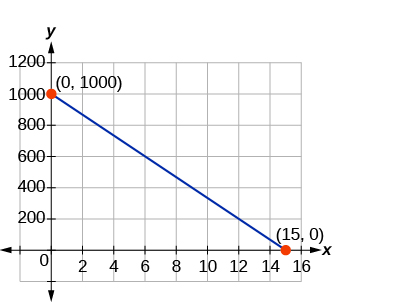Find the $x\text{-}$ and $y\text{-}$ intercepts

Explain what the $x\text{-}$ and $y\text{-}$ intercepts mean for Damien.

(0,1,000),(15,0). At (0,1,000) he left Chicago 0 hours ago and has 1,000 miles left to drive. At (15,0) he left Chicago 15 hours ago and has 0 miles left to drive.

Road trip Ozzie filled up the gas tank of his truck and went on a road trip. The $x\text{-axis}$ on the graph shows the number of miles Ozzie drove since filling up. The $y\text{-axis}$ represents the number of gallons of gas in the truck’s gas tank.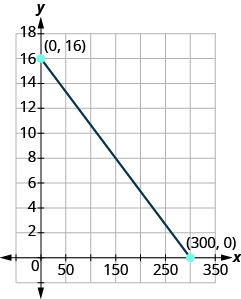Find the $x\text{-}$ and $y\text{-}$ intercepts.

Explain what the $x\text{-}$ and $y\text{-}$ intercepts mean for Ozzie.

Writing exercises

How do you find the $x\text{-intercept}$ of the graph of $3x-2y=6?$

How do you find the $y\text{-intercept}$ of the graph of $5x-y=10?$

Do you prefer to graph the equation $4x+y=-4$ by plotting points or intercepts? Why?

Do you prefer to graph the equation $y=\frac{2}{3}x-2$ by plotting points or intercepts? Why?

Self check

After completing the exercises, use this checklist to evaluate your mastery of the objectives of this section.

What does this checklist tell you about your mastery of this section? What steps will you take to improve?

Is there any normative that regulates the use of silver nanoparticles?
what king of growth are you checking .?
Renato
What fields keep nano created devices from performing or assimulating ? Magnetic fields ? Are do they assimilate ?
why we need to study biomolecules, molecular biology in nanotechnology?
?
Kyle
yes I'm doing my masters in nanotechnology, we are being studying all these domains as well..
why?
what school?
Kyle
biomolecules are e building blocks of every organics and inorganic materials.
Joe
anyone know any internet site where one can find nanotechnology papers?
research.net
kanaga
sciencedirect big data base
Ernesto
Introduction about quantum dots in nanotechnology
what does nano mean?
nano basically means 10^(-9). nanometer is a unit to measure length.
Bharti
do you think it's worthwhile in the long term to study the effects and possibilities of nanotechnology on viral treatment?
absolutely yes
Daniel
how to know photocatalytic properties of tio2 nanoparticles...what to do now
it is a goid question and i want to know the answer as well
Maciej
Abigail
for teaching engĺish at school how nano technology help us
Anassong
Do somebody tell me a best nano engineering book for beginners?
there is no specific books for beginners but there is book called principle of nanotechnology
NANO
what is fullerene does it is used to make bukky balls
are you nano engineer ?
s.
fullerene is a bucky ball aka Carbon 60 molecule. It was name by the architect Fuller. He design the geodesic dome. it resembles a soccer ball.
Tarell
what is the actual application of fullerenes nowadays?
Damian
That is a great question Damian. best way to answer that question is to Google it. there are hundreds of applications for buck minister fullerenes, from medical to aerospace. you can also find plenty of research papers that will give you great detail on the potential applications of fullerenes.
Tarell
what is the Synthesis, properties,and applications of carbon nano chemistry
Mostly, they use nano carbon for electronics and for materials to be strengthened.
Virgil
is Bucky paper clear?
CYNTHIA
carbon nanotubes has various application in fuel cells membrane, current research on cancer drug,and in electronics MEMS and NEMS etc
NANO
so some one know about replacing silicon atom with phosphorous in semiconductors device?
Yeah, it is a pain to say the least. You basically have to heat the substarte up to around 1000 degrees celcius then pass phosphene gas over top of it, which is explosive and toxic by the way, under very low pressure.
Harper
Do you know which machine is used to that process?
s.
how to fabricate graphene ink ?
for screen printed electrodes ?
SUYASH
What is lattice structure?
of graphene you mean?
Ebrahim
or in general
Ebrahim
in general
s.
Graphene has a hexagonal structure
tahir
On having this app for quite a bit time, Haven't realised there's a chat room in it.
Cied
what is biological synthesis of nanoparticles
how did you get the value of 2000N.What calculations are needed to arrive at it
Privacy Information Security Software Version 1.1a
Good
A soccer field is a rectangle 130 meters wide and 110 meters long. The coach asks players to run from one corner to the other corner diagonally across. What is that distance, to the nearest tenths place.
Jeannette has $5 and$10 bills in her wallet. The number of fives is three more than six times the number of tens. Let t represent the number of tens. Write an expression for the number of fives.
What is the expressiin for seven less than four times the number of nickels
How do i figure this problem out.
how do you translate this in Algebraic Expressions
why surface tension is zero at critical temperature
Shanjida
I think if critical temperature denote high temperature then a liquid stats boils that time the water stats to evaporate so some moles of h2o to up and due to high temp the bonding break they have low density so it can be a reason
s.
Need to simplify the expresin. 3/7 (x+y)-1/7 (x-1)=
. After 3 months on a diet, Lisa had lost 12% of her original weight. She lost 21 pounds. What was Lisa's original weight?By Lakeima RobertsBy Jordon Humphreys